# CEOE Middle Level/Intermediate Math: Introduction to Functions Chapter Exam

Exam Instructions:

Choose your answers to the questions and click 'Next' to see the next set of questions. You can skip questions if you would like and come back to them later with the yellow "Go To First Skipped Question" button. When you have completed the practice exam, a green submit button will appear. Click it to see your results. Good luck!

### Page 1

#### Question 3 3. In the figure shown below, the graph of the yellow function is a translation of the graph of the blue function. If the expression for the blue function is y(x) = b(x), which of the following is the equation of the yellow function?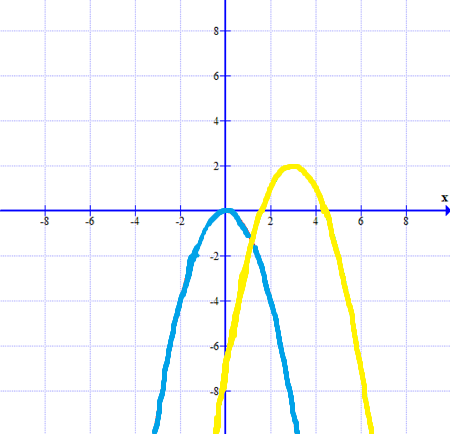#### Question 5 5. Using the function below, evaluate g(7).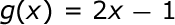### Page 2

#### Question 10 10. What is the domain of the function below?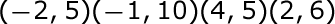### Page 4

#### Question 18 18. Suppose you go to the grocery store and see that apples are on sale for \$0.50 cents each, and you see there are 5 apples left that are available to buy. The amount of money you spend on apples is determined by how many apples you buy, so the amount of money spent on apples is a function of how many apples you buy, where number of apples bought is your input, and money spent on apples is your output. The given function table is used to represent this function. Find the rule, in equation form, for this function table, and fill in the missing entry.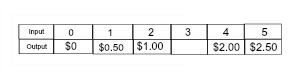### Page 5

#### Question 21 21. Evaluate.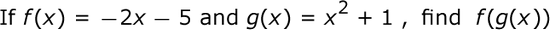### Page 6

#### Question 26 26. If the graph of f(x) is shown below, which choice represents the graph of f(x + 1) + 2?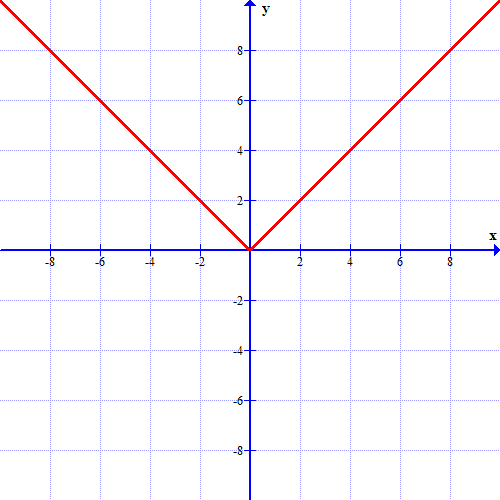#### CEOE Middle Level/Intermediate Math: Introduction to Functions Chapter Exam Instructions

Choose your answers to the questions and click 'Next' to see the next set of questions. You can skip questions if you would like and come back to them later with the yellow "Go To First Skipped Question" button. When you have completed the practice exam, a green submit button will appear. Click it to see your results. Good luck!

Support